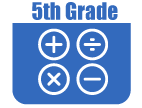IntroductionIn Grade 5, instructional time should focus on three critical areas: (1) developing fluency with addition and subtraction of fractions, and developing understanding of the multiplication of fractions and of division of fractions in limited cases (unit fractions divided by whole numbers and whole numbers divided by unit fractions); (2) extending division to two-digit divisors, integrating decimal fractions into the place value system and developing understanding of operations with decimals to hundredths, and developing fluency with whole number and decimal operations; and (3) developing understanding of volume.

(1) Students will apply their understanding of fractions and fraction models to represent the addition and subtraction of fractions with unlike denominators as equivalent calculations with like denominators. They will develop fluency in calculating sums and differences of fractions, and make reasonable estimates of them. Students will also use the meaning of fractions, of multiplication and division, and the relationship between multiplication and division to understand and explain why the procedures for multiplying and dividing fractions make sense. (Note: This is limited to the case of dividing unit fractions by whole numbers and whole numbers by unit fractions.)

(2) Students will develop understanding of why division procedures work based on the meaning of base-ten numerals and properties of operations. They will finalize fluency with multi-digit addition, subtraction, multiplication, and division. They will apply their understandings of models for decimals, decimal notation, and properties of operations to add and subtract decimals to hundredths. They will develop fluency in these computations, and make reasonable estimates of their results. Students will use the relationship between decimals and fractions, as well as the relationship between finite decimals and whole numbers (e.g., a finite decimal multiplied by an appropriate power of 10 is a whole number), to understand and explain why the procedures for multiplying and dividing finite decimals make sense. They will compute products and quotients of decimals to hundredths efficiently and accurately.

(3) Students will recognize volume as an attribute of three-dimensional space. They will understand that volume can be measured by finding the total number of same-size units of volume required to fill the space without gaps or overlaps. They will understand that a one-unit by one-unit by one-unit cube is the standard unit for measuring volume. They will select appropriate units, strategies, and tools for solving problems that involve estimating and measuring volume. They will decompose three-dimensional shapes and find volumes of right rectangular prisms by viewing them as decomposed into layers of arrays of cubes. They will measure necessary attributes of shapes in order to determine volumes to solve real-world and mathematical problems.The Online Core Resource pages are a collaborative project between the Utah State Board of Education and the Utah Education Network. If you would like to recommend a high quality resource, contact Trish French (Elementary) or Lindsey Henderson (Secondary). If you find inaccuracies or broken links contact resources@uen.org.